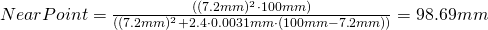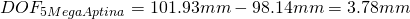# far point

Most distant point on the optical axis with an image of “acceptable sharpness”Where CoC is the Circle of Confusion (the largest accepted Airy-disk) in Millimeter.

Alternatively, we can express the FarPoint using the magnification M :If we use for example a 1/2.5″ 5 Aptina Megapixel greyscale Sensor mit 2.2pixel pitch, we can use the pixel diagonal as CoC for crisp images, sayA 5 Mega lens with f=7.2mm focal length and F-stop F2.4, focused to an object distance of 100mm then has a far point ofund einen Nahpunkt vonand thusIf instead we use a 5 Megapixel greyscale Sony Sensor with 3.45pixel pitch, we can choose as CoC the diagonal of the pixel for crisp images, sayA 5 Mega lens with f=7.2mm focal length and F-stop F2.4, focussed to 100mm then results inmm
und einen Nahpunkt vonthus we getIf we use a color sensor instead we can usefor crisp images. For the two sensors above we then get:To increase the DOF we can increase the Pixel Size, but we either lose resolution, or (at the same pixel count) the magnification changes)
If you change the focal length of a lens in a way, that (with the same sensor) you get the same FOV (then from a different distance) this results in the same DOF !!!
When a lens is focussed to the hyperfocal distance H, the far point is atand the near point is at.
The DOF isthen, thus focussing to the hyperfocal distance results in the largest possible DOF.